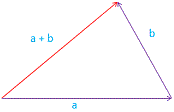#### Vector Calculator

Calculator that allows you to calculate the sum of two dimensional vectors with the entered values (vector coordinates).

 Vector a: Vector b: